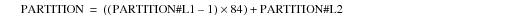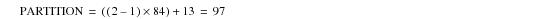# 15.00 - Usage Examples for PARTITION#Ln - Teradata Database

prodname
vrm_release
15.00
category
User Guide
featnum
B035-1094-015K

### Usage Examples for PARTITION#Ln

The following set of sixteen examples provides insight into how you can use the system‑derived PARTITION#Ln set to acquire information about particular partitions in a table that has a multilevel partitioned primary index.

Assume that you have created the orders and lineitem tables defined by the following CREATE TABLE requests.

`     CREATE TABLE orders (`
`       o_orderkey      INTEGER NOT NULL,`
`       o_custkey       INTEGER,`
`       o_orderstatus   CHARACTER(1) CASESPECIFIC,`
`       o_totalprice    DECIMAL(13,2) NOT NULL,`
`       o_orderdate     DATE FORMAT 'yyyy-mm-dd' NOT NULL,`
`       o_orderpriority CHARACTER(21),`
`       o_comment       VARCHAR(79))`
`     PRIMARY INDEX (o_orderkey)`
`     PARTITION BY (`
`       RANGE_N(o_custkey   BETWEEN 0 `
`                           AND 49999 `
`                           EACH  100),`
`       RANGE_N(o_orderdate BETWEEN DATE '2000-01-01' `
`                           AND     DATE '2006-12-31'`
`                           EACH INTERVAL '1' MONTH))`
`     UNIQUE INDEX (o_orderkey);`
` `
`     CREATE TABLE lineitem (`
`       l_orderkey      INTEGER NOT NULL,`
`       l_partkey       INTEGER NOT NULL,`
`       l_suppkey       INTEGER,`
`       l_linenumber    INTEGER,`
`       l_quantity      INTEGER NOT NULL,`
`       l_extendedprice DECIMAL(13,2) NOT NULL,`
`       l_discount      DECIMAL(13,2),`
`       l_tax           DECIMAL(13,2),`
`       l_returnflag    CHARACTER(1),`
`       l_linestatus    CHARACTER(1),`
`       l_shipdate      DATE FORMAT 'yyyy-mm-dd',`
`       l_commitdate    DATE FORMAT 'yyyy-mm-dd',`
`       l_receiptdate   DATE FORMAT 'yyyy-mm-dd',`
`       l_shipinstruct  VARCHAR(25),`
`       l_shipmode      VARCHAR(10),`
`       l_comment       VARCHAR(44))`
`     PRIMARY INDEX (l_orderkey)`
`     PARTITION BY (`
`       RANGE_N(l_suppkey  BETWEEN 0 `
`                          AND  4999 `
`                          EACH   10),`
`       RANGE_N(l_shipdate BETWEEN DATE '2000-01-01' `
`                          AND     DATE '2006-12-31' `
`                          EACH INTERVAL '1' MONTH));`

### Example

The following example selects all rows from the orders table from January, 2000 (`orders.PARTITION#L2=1`).

`     SELECT * `
`     FROM orders `
`     WHERE orders.PARTITION#L2 = 1;`

Because PARTITION#L2 is not referenced in the select list, its value is not returned.

Because only one table is referenced by the request, you need not fully qualify PARTITION#L2 in the WHERE clause, though this example does so.

### Example

The following example selects all rows from the orders table for customers that meet the following criteria:

• custkey values are between 100 and 199, specified by partition number 2 for level 1 (`WHERE orders.PARTITION#L1=2`)
• orderdate values are from January, 2001, specified by partition number 13 for level 2
(`AND orders.PARTITION#L2=13`)
• `     SELECT * `
`     FROM orders `
`     WHERE orders.PARTITION#L1 =  2 `
`     AND   orders.PARTITION#L2 = 13;`

Because neither PARTITION#L1 nor PARTITION#L2 is referenced in the select list, their values are not returned.

Because only one table is referenced by the request, you need not fully qualify PARTITION#L1 and PARTITION#L2 in the WHERE clause, though this example does so.

### Example

The following example selects all rows from the orders table for customers that meet the following criteria:

• custkey values between 100 and 199, specified by partition number 2 for level 1
• orderdate values from January, 2001, specified by partition number 13 for level 2
• Note that the results set returned by this request is identical to that returned by the query in “Example 2” on page 813.

Teradata Database derives these predicates from the combined partitioning expression, which is expressed by the clause `WHERE orders.PARTITION=97` in the request.

For this request, the partition number for the combined partitioning expression is derived from the following calculation:where:

 Equation element … Specifies … PARTITION the partition number for the combined partitioning expression. PARTITION#L1 the partition number for level 1 rows containing custkey values in the inclusive range 100 - 199. 84 the number of partitions defined for level 2 of the orders table by the following partitioning expression: `   RANGE_N(o_orderdate BETWEEN DATE '2000-01-01'` `                       AND DATE '2006-12-31'` `                       EACH INTERVAL '1' MONTH))` which defines six complete years, each having twelve months. `     6 x 12 = 84` PARTITION#L2 the partition number for level 2 rows containing orderdates from January, 2001, which is 13.

The result is the partition number for the combined partitioning expression:` `
`     SELECT * `
`     FROM orders `
`     WHERE orders.PARTITION = 97;`

Because you do not specify PARTITION explicitly in the select list, the request does not return its value. Because only one table is referenced by the request, you need not fully qualify PARTITION in the WHERE clause, though this example does so.

### Example

In the following example, you must qualify PARTITION#L2 in the WHERE clause predicate specification because you reference it in both the orders and the lineitem tables:

`     SELECT * `
`     FROM orders, lineitem `
`     WHERE orders.PARTITION#L2 = 3 `
`     AND   lineitem.PARTITION#L2 = 5 `
`     AND   orders.o_orderkey = lineitem.l_orderkey;`

Because you do not specify either orders, PARTITION#L2, or orders.PARTITION#L2 in the select list, the request does not return their values.

### Example

The following example aborts and returns and error message because you cannot specify the PARTITION#Ln keyword in a VALUES clause:

`     INSERT INTO orders `
`     VALUES (PARTITION#L1, 10, 'A', 599.99, DATE '2006-02-07',`
`            'HIGH', 'Invalid insert');`

### Example

The following example returns a list of all the populated partitions for level 2 of the orders table:

`     SELECT DISTINCT CAST (PARTITION#L2 AS BYTEINT) `
`     FROM orders `
`     ORDER BY PARTITION#L2;`

Teradata Database selects the partition number for level 2 from each row in the partition, but because of the DISTINCT operator, it reports only one row for the partition. The system does not return any rows for empty partitions.

Because only one table is referenced by the request, you need not fully qualify PARTITION#L2 in either the select list or the ORDER BY clause.

### Example

Consider the following view definition:

`     CREATE VIEW ordersv AS `
`       SELECT * `
`       FROM orders;`

Because they are not explicitly selected in the view definition, you cannot select any of the system‑derived PARTITION columns in the orders table through it.

For example, all three of the following SELECT requests fail with an Invalid Partition field error:

`     SELECT PARTITION `
`     FROM ordersv; -- 5879 Invalid Partition field.`
` `
`     SELECT PARTITION#L1 `
`     FROM ordersv; -- 5879 Invalid Partition field.`
` `
`     SELECT PARTITION#L5 `
`     FROM ordersv; -- 5879 Invalid Partition field.`

Now consider the following view definition that does explicitly specify the system‑derived column PARTITION#L1:

`     CREATE VIEW ordersvp AS `
`       SELECT orders.*, PARTITION#L1 `
`       FROM orders;`

If you want to select from the orders table using this view, you can submit a `SELECT *` request, and it returns the value of the system‑derived PARTITION#L1 column as well as the values for the physical columns defined for the table.

Similarly, you can select only the system‑derived PARTITION#L1 column using this view.

### Example

The outcome of the following request depends on whether the relation defined by the view is populated or empty, as follows:

 IF the orders table has … THEN the query returns … any rows that satisfy the ordersvp view definition the maximum partition number in the view for level 1 of the orders table. no rows that satisfy the ordersvp view definition null.
`     SELECT MAX(PARTITION#L1) `
`     FROM ordersvp; `

### Example

Because it is not explicitly selected in the view definition, you cannot select the system‑derived combined PARTITION column in the orders table using the ordersvp view.

The following SELECT request fails with an Invalid Partition field error:

`     SELECT PARTITION `
`     FROM ordersvp;    -- 5879 Invalid Partition field.`

### Example

This example fails with the same error as the query in “Example 9” on page 816 because the system‑derived partition column PARTITION#L5 is not specified explicitly in the definition of the ordersvp view.

`     SELECT PARTITION#L5 `
`     FROM ordersvp; -- 5879 Invalid Partition field.`

### Example

In the following view definition, the system-derived column PARTITION#L1 is explicitly selected and then redefined, or aliased, as P#1.

If you select all columns from the P#1 view by specifying an ASTERISK character as the only entry in the select list, then the system returns the value of PARTITION#L1 as P#1, but it does not return PARTITION#L1 as a column.

Given this view definition, you can also explicitly select P#1, but not PARTITION#L1, through it.

`     CREATE VIEW ordersvp1 AS `
`     SELECT orders.*, PARTITION#L1 AS P#1 `
`     FROM orders;`

### Example

The outcome of the following request depends on whether the relation defined by the view is populated or empty, as follows.

 IF the orders table has … THEN the query returns … any rows that satisfy the ordersvp1 view definition the minimum partition number for level 1. no rows that satisfy the ordersvp1 view definition null.
`     SELECT MIN(P#1) `
`     FROM ordersvp1;`

### Example

The following requests against the ordersvp1 view all fail with an Invalid Partition field error because they specify a name for a system‑derived partition column in their select list that is not defined explicitly in the view definition.

The following request fails because PARTITION is not defined explicitly in ordersvp1:

`     SELECT PARTITION `
`     FROM ordersvp1; -- 5879 Invalid Partition field.`

The following request fails because PARTITION#L1 is not defined explicitly in ordersvp1; it is aliased as P#1.

`     SELECT PARTITION#L1 `
`     FROM ordersvp1; -- 5879 Invalid Partition field.`

To obtain the desired result of the request, you need to rewrite is as follows:

`     SELECT P#1`
`     FROM ordersvp1;`

The following request fails because PARTITION#L5 is not defined explicitly in ordersvp1:

`     SELECT PARTITION#L5 `
`     FROM ordersvp1; -- 5879 Invalid Partition field.`

### Example

The outcome of the following request depends on whether the table is populated or empty, as follows:

 IF the orders table is … THEN the query returns … populated the number of partitioning levels defined for the table. Equivalently, the number of partitioning expressions defined for the table. These are just two different ways of saying the same thing. not populated null.
`     SELECT MAX(CASE_N(PARTITION#L1=0,  PARTITION#L2=0, PARTITION#L3=0,`
`                       PARTITION#L4=0,  PARTITION#L5=0, PARTITION#L6=0, `
`                       PARTITION#L7=0,  PARTITION#L8=0, PARTITION#L9=0,`
`                       PARTITION#L10=0, PARTITION#L11=0,`
`                       PARTITION#L12=0, PARTITION#L13=0,`
`                       PARTITION#L14=0, PARTITION#L15=0, NO CASE)) - 1 `
`     FROM orders;`

Note that if orders had more than 65,535 combined partitions, you would need to specify 62 partition numbers (PARTITION#L1 through PARTITION#L62) rather than 15.